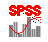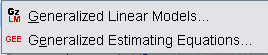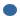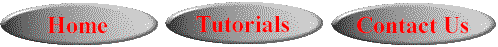# SPSS On-Line Training WorkshopHOME Table of Contents Data Editor Window Syntax  Editor WindowCarl Lee Felix Famoye About Us Chart Editor Window Output Window Overview of Data Analysis Manipulation of Data Analysis of Data Projects & Data Sets Integrate R into SPSS

# Generalized Linear ModelIn this Tutorial:

Generalized
Linear Models

Generalized
Estimating Eq.

Analysis of DataGeneralized Linear Model menu includes techniques of Generalized Linear Models and Generalized Estimating Equations. This page demonstrates how to apply the Generalized Linear Models only. Users who are interested in the Generalized Estimating Equation technique should consult the SPSS Help menu.

Click on the following movie clip to learn the Generalized Linear Model Technique.

In this on-line workshop, you will find many movie clips. Each movie clip will demonstrate some specific usage of SPSS.

Generalized Linear Models refer to the models involving link functions. This is an extension of general linear model so that a dependent variable can be linearly related to factors and/or covariates by using a link function.. The dependent variable does not require normal assumption. The dependent variable could be count (as in Poisson regression model or negative binomial regression model) or ordinal (as in logistic regression model). The binary logistic regression is a generalized linear model having a logit link function acting on a binary response variable.

The main dialog box for this technique has the following taps at the top:Type of Model: Users select the type of response to be analyzed. This technique allows for scale response, ordinal response, count data, binary response and mixture data. Here you select the model type, e.g. “Poisson loglinear” for Poisson regression model. One can do multiple linear regression by selecting Linear under the scale Response. You can select binary logistic and a custom model. Among the custom models are normal, binomial and negative binomial. However, note that SPSS does not estimate the scale parameter under the negative binomial model.Response: This  menu asks for dependent variable and scale weight (if any). Select your dependent variable. If the response variable is binary, you can specify the reference category. You can also specify a binary response in the form of “n” and “y”, where “n” is the number of trials and “y” is the number of “events” or “successes”.Predictors: This menu asks for factors and covariates to be used as independent variables. Select the factors, covariates and any offset variable you may have. “Options” in this submenu will allow you to specify how to handle missing values and how to order the factors.Model: This menu asks users to define the model. Specify the model. The default model is not the full factorial. It is the intercept-only model. You must specify the model terms explicitly.Estimation: This allows users to decide how the model parameters are to be estimated, and how the covariance matrix required for the modeling to be estimated. You can also make changes to the default settings for the iterations.Statistics: Users can choose a variety of statistics to be reported in the output. By default, Type III sum of squares are computed. You can change this.EM Means: This tab will allow users to display the estimated marginal means for the factor levels.Save: Users can save various statistics for further analysis and model diagnostics.Export: Users can export the final model as data or as XML.

In the following movie clip we will demonstrate how to use the Generalized Linear Model procedure. We will apply a log-linear Poisson regression model to fit a count dataset. For a log-linear Poisson regression model, Y is a count response variable with a Poisson distribution. The mean of Y, m, depends on k-1 predictors. C is a measure of exposure and it is called an offset variable. In some applications it is one. Since we are interested in the log-linear function, we will use  m = C*exp(X'b) and if we took the log of this we would get a linear function.

The data set used for this demonstration is the Fabric data set (See the Data Set page for details).  The dependent (or response) variable ‘faults’ is the number of faults in a roll of fabric and the predictor variable ‘log_length’ is the logarithm of the length of the roll. Under the log-linear mean specification, the Poisson regression model will be applied to fit the data.

Generalized Estimating Equations

This technique extends the generalized linear model to include analysis of repeated measurements or other correlated observations. For example, it allows for within-subject covariates or auto-correlated response data, such as autoregressive data. The possible types of response variables are the same as the generalized linear models the response variable involve link functions. Like the generalized linear models procedure, the main dialog box has tabs at the top. The first tab at top is “Repeated”. In repeated tab, specify the subject variables and within-subject variables and the structure of working correlation matrix. There is no movie clip developed for this procedure. Users need to refer to the SPSS Help menu for more information.©This online SPSS Training Workshop is developed by Dr Carl Lee, Dr Felix Famoye and  Barbara Shelden, student assistant, Department of Mathematics, Central Michigan  University. All rights reserved.